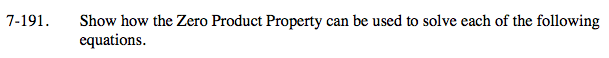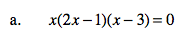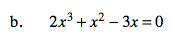Home > A2C > Chapter 7 > Lesson 7.3.3 > Problem7-191

7-191.
1. Show how the Zero Product Property can be used to solve each of the following equations. Homework Help ✎

1. x(2x − 1)(x − 3) = 0

2. 2x3 + x2 − 3x = 0x = 0, 0.5, 3The factored form is x(x − 1)(2x + 3) = 0